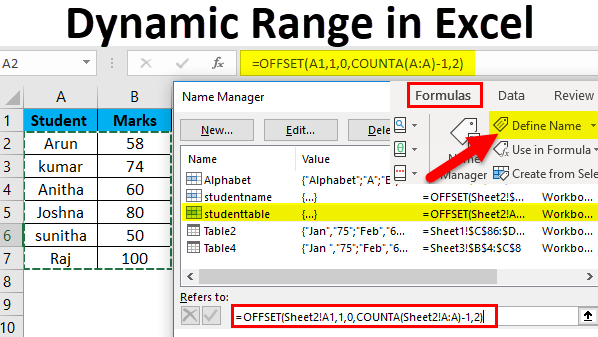# How To Use VBA Dynamic Range In Excel

Oct 23, 2020 • edited Oct 24, 2020Below we will look at a program in Excel VBA that colors the maximum value of a dynamic range.

Situation:

Each time we add a number and we click the command button, we want Excel VBA to color the maximum value of these numbers.Place a command button on your worksheet and add the following code lines:

1. First, we declare one variable and two Range objects. One variable of type Double we call maximum. We call the Range objects rng and cell.

``````          Dim maximum As Double, rng As Range, cell As Range
``````

2. We add the line which changes the background color of all cells to ‘No Fill’.

``````          Cells.Interior.ColorIndex = 0
``````

3. We initialize rng with the numbers. We use the CurrentRegion property for this. CurrentRegion is useful when we don't know the exact boundaries of a range in advance.

``````          Set rng = Range(&quot;A1&quot;).CurrentRegion
``````

4. We initialize maximum with the maximum value of the numbers. We use the worksheet function Max to find the maximum value.

``````          maximum = WorksheetFunction.Max(rng)
``````

5. Finally, we color the maximum value. We use a For Each Next Loop.

``````          For Each cell In rng

If cell.Value = maximum Then cell.Interior.ColorIndex = 22
``````

Next cell

Note: instead of ColorIndex number 22 (red), you can use any ColorIndex number.